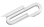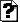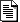Spring 2018

# computational

## Chris

I'm having some trouble getting the discrete transform to look as it should, but here's what I have so far (the notebook is attached).

## Jim

I think this is close, though I haven't looked closely enough at your definitions - we should discuss.

• Part of the advantage of numpy is that it lets you do math on arrays without loops. I don't think you need the "for" loop in inner; just turn x into an numpy array and then do the formulat to it.
• Starting with an input which isn't symmetric around x=0 will give you an extra phase ripple - that may be part of what you're not expecting.
• The output Cj will have frequencies k=0 at i=0, k=1 at i=1 and i=-1 , k=2 at i=2 and i=-2, and so on. So to get the 0 on the graph to mean 0 frequency, the output needs to be reordered, something like np.concatenate(Cj[N/2+1:0]
• Typically you'll want to plot power, i.e. sqrt(|Cj|**2).

Talk to you tomorrow.

We should do a tiny (like N=4) example with some dirt simple (like y=[1,1,0,0] or [1,-1,1,-1] or [1,1,1,1]) inputs to see if we make sense of those cases. That should make it clearer what the output k=0...(N-1) components mean.

## Jim in class

I showed you two more notebook files: fourier.ipynb (looking at the N=4 case , plotting the basis functions, calculating the fourier coefficients as a dot products) and fourier_128_tophat (just the transform with N=128 for a "tophat" function __--__ .)

The .ipynb and .html files are attached.

## attachmentslast modified sizeWeek1.ipynb Wed Nov 29 2023 06:32 am 37KWeek1_jim.html Wed Nov 29 2023 06:32 am 277KWeek1_jim.ipynb Wed Nov 29 2023 06:32 am 23Kfourier.html Wed Nov 29 2023 06:32 am 328Kfourier.ipynb Wed Nov 29 2023 06:32 am 69Kfourier_128_tophat.html Wed Nov 29 2023 06:32 am 294Kfourier_128_tophat.ipynb Wed Nov 29 2023 06:32 am 35K